Practice the AP 7th Class Maths Bits with Answers 10th Lesson Construction of Triangles on a regular basis so that you can attempt exams with utmost confidence.

## AP Board 7th Class Maths Bits 10th Lesson Construction of Triangles

Multiple Choice Questions :

Question 1.
Which of the following measurements are correct to construct a triangle?
(A) 2 cm, 3 cm, 5 cm
(B) 4 cm, 5 cm, 7 cm
(C) 1 cm, 2 cm, 3 cm
(D) 5 cm, 6 cm, 13 cm
(B) 4 cm, 5 cm, 7 cm

Question 2.
If a, b and c are the lengths of sides of a triangle, then which of the following is true?
(A) a – b > c
(B) a + b < c
(C) b + c > a
(D) c – a = b
(C) b + c > a

Question 3.
Which measurements are suitable for the construction of a right-angled triangle?
(A) 2 cm, 3 cm, 4 cm
(B) 7 cm, 4 cm, 5 cm
(C) 9 cm, 7 cm, 10 cm
(D) 3 cm, 4 cm, 5 cm
(D) 3 cm, 4 cm, 5 cmQuestion 4.
In which of the following situation ∆ABG is constructed?
(A) AB + BC = AC
(B) AB + BC ≯ AC
(C) BC + CA < AB
(D) AB + AC > BC
(D) AB + AC > BC

Question 5.
Which of the following measurements are not able to construct a triangle?
(A) AB = 5 cm, ∠A = 105b, ∠B = 95°
(B) 3 cm, 3 cm, 3 cm
(C) ∠P = 60°, PQ = 8 cm, ∠Q = 70°
(D) AD = 4 cm, ∠D = 90°, DI = 4 cm
(A) AB = 5 cm, ∠A = 105b, ∠B = 95°

Question 6.
A triangle has _______
(A) one right angle
(B) two obtuse angles
(C) two right angles
(D) one right angle and one obtuse angle
(A) one right angle

Question 7.
A triangle can be drawn in which of the following situations? When _______
(A) three sides of the triangle are given.
(B) three angles are given.
(C) three acute angles are given.
(D) three acute one obtuse angles are given.
(A) three sides of the triangle are given.

Question 8.
In ∆PQR, ∠R = 90°, then its hypotenuse is _______
(A) PQ
(B) QR
(C) PR
(D) None
(A) PQ

Question 9.
In ∆ADI, AD = DI, then it is a type of triangle.
(A) Scalene
(B) Isosceles
(C) Equilateral
(D) Right-angled triangle
(B) Isosceles

Question 10.
In ∆XYZ, XY == YZ = 6 cm, ∠Y = 90°, then it is a ______ triangle.
(A) Isosceles
(B) Right-angled
(C) Right-angled isosceles
(D) Equilateral
(C) Right-angled isosceles

Question 11.
In which of the following situation ∆XYZ is not constructed?
(A) XY + YZ > XZ
(B) XY + XZ > YZ
(C) XY < XZ + ZY
(D) XY > XZ + ZY
(D) XY > XZ + ZY

Question 12.
Which measurements are able to construct a triangle?
(A) 4 cm, 7 cm, 11 cm
(B) 2 cm, 3 cm, 6 m
(C) 5 cm, 6 m, 8 m
(D) 9 cm, 2 cm, 5 cm
(C) 5 cm, 6 m, 8 m

Question 13.
Which of the following measurements are not able to construct a triangle?
(A) 2 cm, 3 cm, 4 cm
(B) 11 cm, 7 cm, 18 cm
(C) 7 cm, 4 cm, 5 cm
(D) 9 cm, 7 cm, 10 cm
(B) 11 cm, 7 cm, 18 cm

Question 14.
In which of the following measurements a triangle is constructed?
(A) ∆NET, NE= 6.4 cm, ∠N = 80°, ∠E = 100°
(B) ∆XYZ, XY = 2 cm, YZ = 8 cm, XZ = 4 cm
(C) ∆RUN, RN = 5 cm, ∠R = ∠N = 45°
(D) ∆ABC, IB = 90°, AC = 7 cm, AB = 8 cm.
(C) ∆RUN, RN = 5 cm, ∠R = ∠N = 45°Question 15.
A triangle can be drawn in which of – the following situations? When _______
(A) three sides of the triangle are given
(B) three angles are given
(C) three acute angles are given
(D) three acute one obtuse angles are given
(A) three sides of the triangle are given

Question 16.
A triangle can’t be drawn in which of the following situations? When _______
(A) three sides are given
(B) two sides and the angle included between them.
(C) two angles and the side included between them.
(D) two interior angles and one obtuse angle
(D) two interior angles and one obtuse angle

Question 17.
Number of individual measurements are required to construct 4n equilateral triangle?
(A) 1
(B) 2
(C) 3
(D) 4
(A) 1

Question 18.
Number of individual measurements are required to construct a triangle?
(A) 1
(B) 2
(C) 3
(D) 4
(C) 3

Question 19.
In ∆AN, $$\overline{\mathrm{MA}}=\overline{\mathrm{AN}}$$ then It Is a type of triangle.
(A) Scalene
(B) Isosceles
(C) Equilateral
(D) Right-angled triangle
(B) Isosceles

Question 20.
In which case it is not possible to construct a triangle, with the given measures of sides in cm?
(A) 3, 4, 5
(B) 4, 4, 8
(C) 3, 5, 7
(D) 7, 7, 7
(B) 4, 4, 8

Reasoning Questions :

Find the number of triangles in the given figures.

Question 1.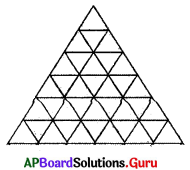(A) 87
(B) 78
(C) 67
(D) 68
(B) 78

Question 2.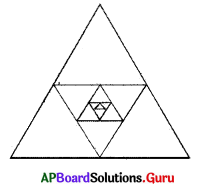(A) 41
(B) 31
(C) 21
(D) 11
(C) 21Question 3.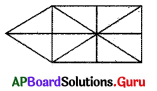(A) 21
(B) 23
(C) 22
(D) 24
(A) 21

Question 4.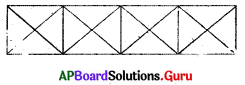(A) 37
(B) 36
(C) 38
(D) 35
(C) 38

Question 5.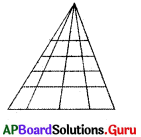(A) 40
(B) 30
(C) 50
(D) 60
(C) 50

Question 6.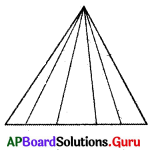(A) 5
(B) 15
(C) 25
(D) 35
(B) 15

Question 7.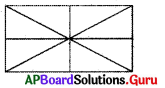(A) 16
(B) 15
(C) 18
(D) 17
(A) 16

Fill In the blanks:

Question 1.
Number of individual measurements required to construct an equilateral triangle _______________ .
1

Question 2.
Least number of independent measurements required to construct a right angled triangle ______________ .
2

Question 3.
Triangle can have _______________ right angles.
1

Question 4.
If ∠B = 90° in MBC, then hypotenuse is ______ .
AC

Question 5.
If ∠P = 30° and ∠Q = 70° in ∆PQR, then ∠R = ______
80°

Question 6.
If in ∆XYZ. XY = YZ = 7cm. then ∆XYZ is _______ triangle.
Isosceles triangleQuestion 7.
A triangle can have _______ obtuse angles.
1

Question 8.
Triangle can be constructed with at least _______ acute angles.
2

Question 9.
7 cm. 24 cm, 25 cm are the sides of _______ triangle.
Right-angled triangle

Question 10.
If any two sides of a triangle are equal, then the ______ triangle can form.